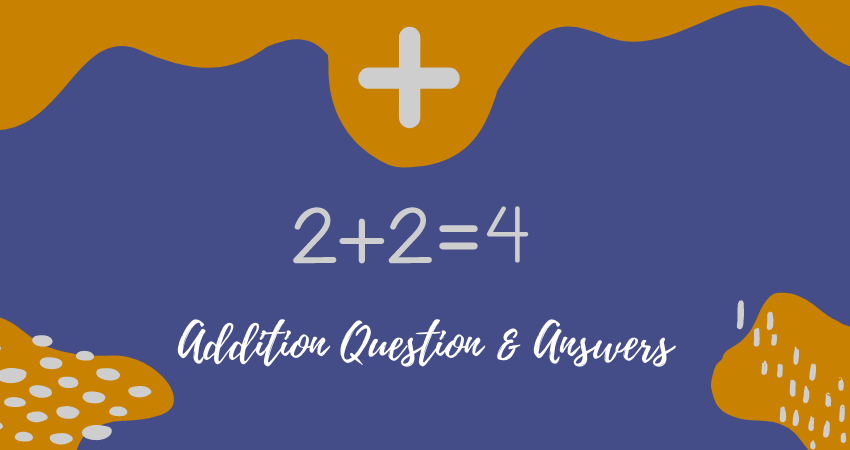Online Addition Questions with Answers | Best Kids Learning Platform - Easyshiksha• 1+2 = ?
• 4+5 = ?
• 3+5 = ?
• 2+6 = ?
• 3+7 = ?
• 7+5 = ?
• 8+6 = ?
• 9+7 = ?
• 8+9 = ?
• 9+9 = ?
• 11+13 = ?
• 10+12 = ?
• 13+14 = ?
• 15+11 = ?
• 20+40 = ?
• 10+10 = ?
• 100+20 = ?
• 3+5 = ?
• 640+100 = ?
• 63+98 = ?